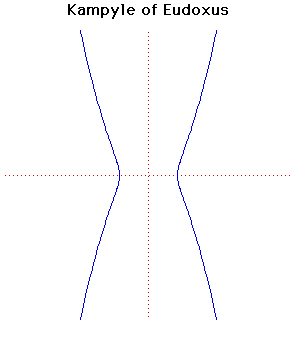# Curves

### Kampyle of EudoxusCartesian equation:
$a^{2}x^{4} = b^{4}(x^{2} + y^{2})$
Polar equation:
$r = b^{2}/(a \cos^{2}( \theta ))$

### Description

A curve studied by Eudoxus also in relation to the classical problem of duplication of the cube.

Eudoxus was a pupil of Plato. His main work was in astronomy. He was the first to describe the constellations and invented the astrolabe. He introduced the study of mathematical astronomy into Greece.

Eudoxus found formulas for measuring pyramids cones and cylinders. His work contains elements of the calculus with a rigorous study of the method of exhaustion.

The word kampyle comes from the Greek καμπύλη meaning curve.

Further information:

J H McCoy

### Associated Curves

Definitions of the Associated curves